# February 2020For this month’s Traders’ Tips, the focus is John Ehlers’ article in this issue, “Reflex: A New Zero-Lag Indicator.” Here, we present the February 2020 Traders’ Tips code with possible implementations in various software.

You can right-click on any chart to open it in a new tab or window and view it at it’s originally supplied size, often much larger than the version printed in the magazine.

The Traders’ Tips section is provided to help the reader implement a selected technique from an article in this issue or another recent issue. The entries here are contributed by software developers or programmers for software that is capable of customization.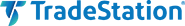In “Reflex: A New Zero-Lag Indicator” in this issue, author John Ehlers introduces a new averaging indicator that he has designed with reducing lag in mind. According to the author, this new indicator can be used to generate signals in a more timely manner than other lagging calculations.

In his article, Ehlers provides TradeStation EasyLanguage code for the indicators. Here, we are providing EasyLanguage functions for the reflex and trendflex indicators that you can use in developing your own indicators and strategies. We’ve also provided an example strategy to help you get started. The EasyLanguage code is shown here and can also be downloaded by visiting our TradeStation and EasyLanguage support forum at the link provided after the code listing.

```Function: _Reflex

{
TASC Feb 2020
(C) 2019 John F. Ehlers
_Reflex function
}
Inputs:
Length(numericsimple);
Vars:
Slope( 0 ),
sum( 0 ),
count( 0 ),
a1( 0), b1(0), c1(0),
c2(0), c3(0), Filt(0),
MS(0),Reflex(0);

//Gently smooth the data in a SuperSmoother
a1 = ExpValue( -1.414 * 3.14159 /
( .5 * Length ) ) ;
b1 = 2 * a1 * Cosine( 1.414 * 180 /
( .5 * Length ) ) ;
c2 = b1 ;
c3 = -a1 * a1 ;
c1 = 1 - c2 - c3 ;
Filt = c1 * ( Close + Close ) / 2
+ c2 * Filt + c3 * Filt ;

//Length is assumed cycle period
Slope = ( Filt[Length] - Filt ) / Length ;

//Sum the differences
Sum = 0;
for count = 1 to Length
begin
Sum = Sum + ( Filt + count * Slope ) -
Filt[count] ;
end;
Sum = Sum / Length ;

//Normalize in terms of Standard Deviations
MS = .04 * Sum * Sum + .96 * MS ;
if MS <> 0 then
Reflex = Sum / SquareRoot( MS ) ;

_Reflex = Reflex ;

Function: _TrendFlex

{
TASC Feb 2020
(C) 2019 John F. Ehlers
_Trendflex function
}
inputs:
Length( numericsimple ) ;
Vars:
Sum( 0 ), Count( 0 ), a1( 0 ),
b1( 0 ), c1( 0 ), c2( 0 ), c3( 0 ),
Filt( 0 ), MS( 0 ), Trendflex( 0 ) ;

//Gently smooth the data in a SuperSmoother
a1 = ExpValue( -1.414 * 3.14159 /
( .5 * Length ) ) ;
b1 = 2 * a1 * Cosine( 1.414 * 180 /
( .5 * Length ) ) ;
c2 = b1 ;
c3 = -a1 * a1 ;
c1 = 1 - c2 - c3 ;
Filt = c1 * ( Close + Close ) / 2 +
c2 * Filt + c3 * Filt ;

//Sum the differences
Sum = 0 ;
for count = 1 to Length
begin
Sum = Sum + Filt - Filt[count] ;
end ;
Sum = Sum / Length ;

//Normalize in terms of Standard Deviations
MS = .04 * Sum * Sum + .96 * MS ;
if MS <> 0 then
Trendflex = Sum / SquareRoot( MS ) ;

_Trendflex = trendflex ;

Indicator:  Trendflex Cross

{
TASC Feb 2020
(C) 2019 John F. Ehlers
}
inputs:
FastLength( 20 ),
SlowLength( 50 ) ;
variables:
FastTF( 0 ),
SlowTF( 0) ;

FastTF = _TrendFlex( FastLength ) ;
SlowTF = _TrendFlex( SlowLength ) ;

Plot1( FastTF - SlowTF ) ;
Plot2( 0 ) ;

Strategy: Trendflex Cross

{
TASC Feb 2020
(C) 2019 John F. Ehlers
}
inputs:
FastLength( 20 ),
SlowLength( 50 ) ;
variables:
FastTF( 0 ),
SlowTF( 0) ;

FastTF = _TrendFlex( FastLength ) ;
SlowTF = _TrendFlex( SlowLength ) ;

if FastTF crosses over SlowTF then
else if FastTF crosses under SlowTF then
SellShort next bar at Market ;
```

A sample chart is shown in Figure 1.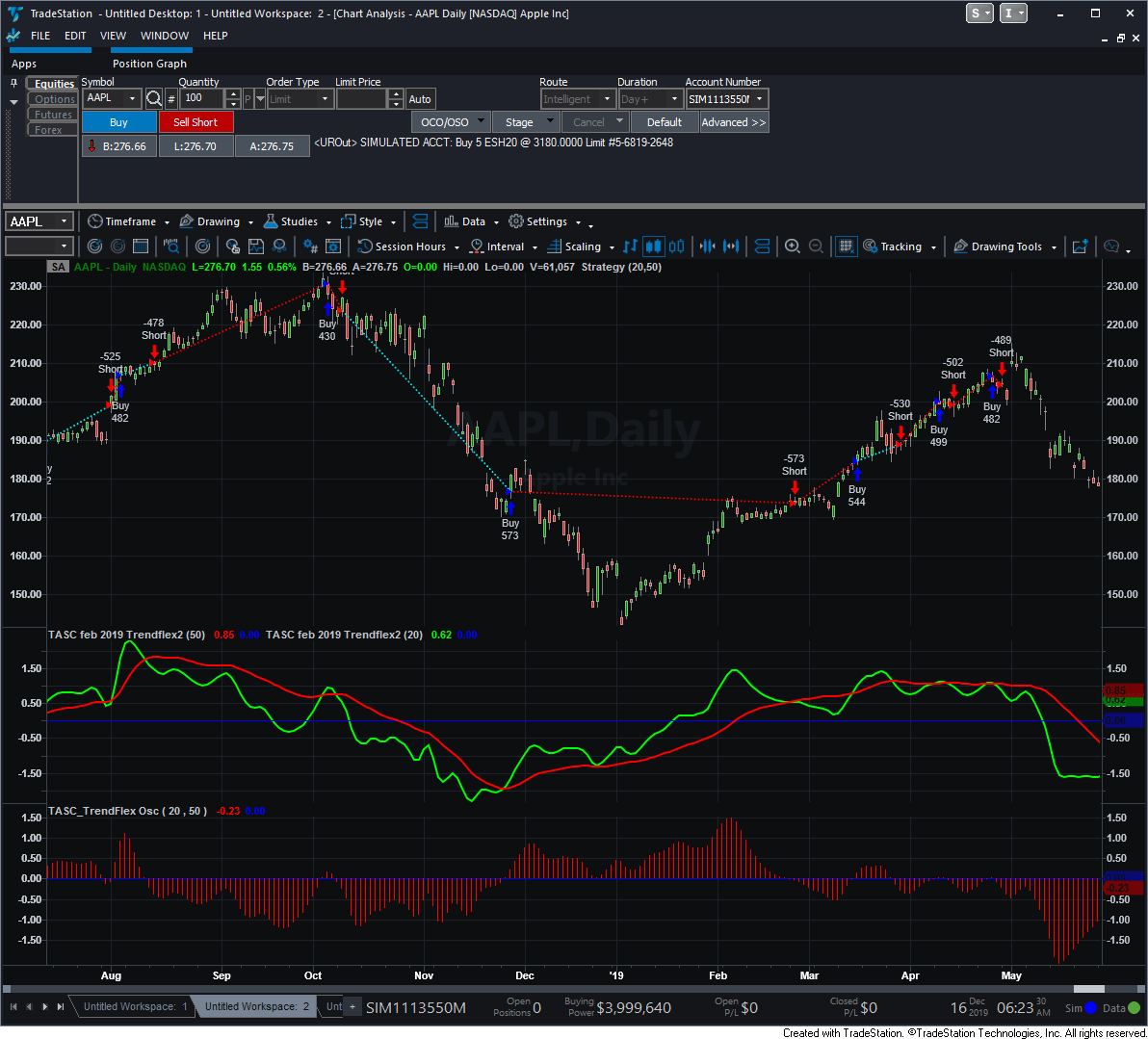FIGURE 1: TRADESTATION. This shows an example of the trendflex indicator and strategy applied on a daily TradeStation chart of AAPL.

—Doug McCrary

BACK TO LIST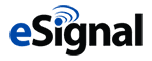### eSIGNAL: FEBRUARY 2020

For this month’s Traders’ Tip, we’ve provided the study ReflexIndicators.efs based on the article by John Ehlers in this issue, “Reflex: A New Zero-Lag Indicator.” The study displays both the reflex and trendflex indicators discussed in the article.

The studies contain formula parameters that may be configured through the edit chart window (right-click on the chart and select “edit chart”). A sample chart is shown in Figure 2.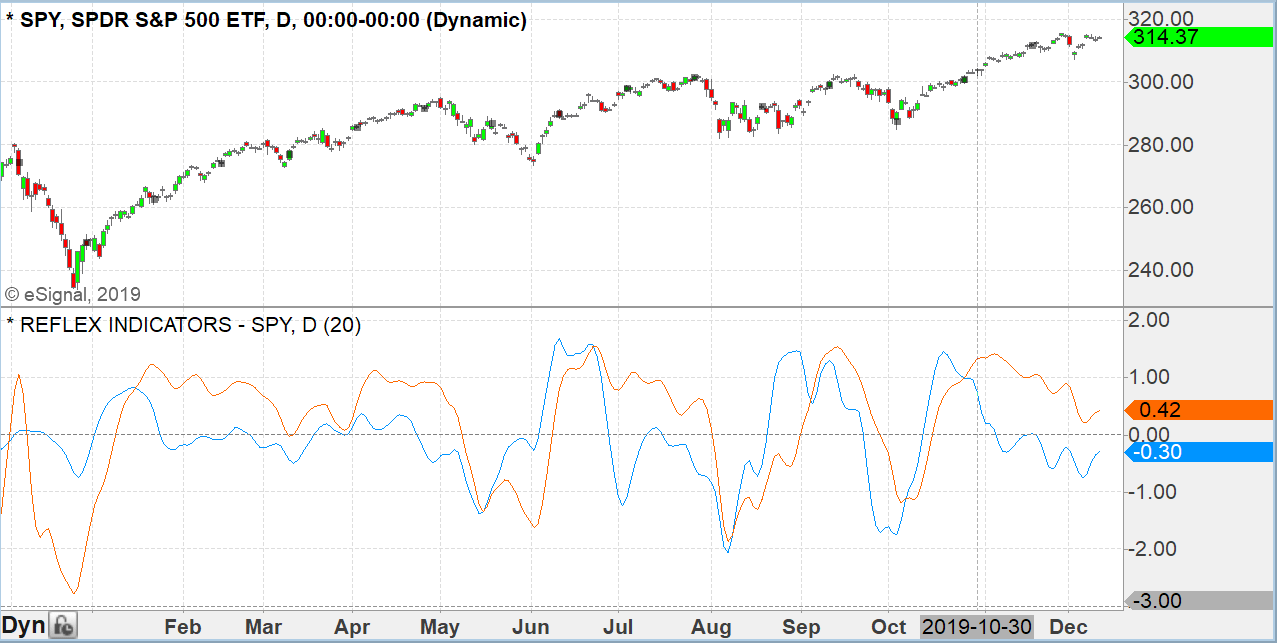FIGURE 2: ESIGNAL. Here is an example of the studies plotted on a daily chart of SPY.

To discuss this study or download a complete copy of the formula code, please visit the EFS library discussion board forum under the forums link from the support menu at www.esignal.com or visit our EFS KnowledgeBase at http://www.esignal.com/support/kb/efs/. The eSignal formula script (EFS) is also available for copying & pasting here:

```/**********************************
Provided By:
eSignal is a service mark and/or a registered service mark of Intercontinental Exchange, Inc.
in the United States and/or other countries. This sample eSignal Formula Script (EFS)
is for educational purposes only.
Intercontinental Exchange, Inc. reserves the right to modify and overwrite this EFS file with each new release.

Description:
Reflex: A New
Zero-Lag Indicator
by John F. Ehlers

Version:            1.00  12/10/2019

Formula Parameters:                     Default:
Length                                  20

Notes:
The related article is copyrighted material. If you are not a subscriber

**********************************/
var fpArray = new Array();
var bInit = false;

function preMain() {
setStudyTitle("REFLEX INDICATORS");
setCursorLabelName("Reflex Indicator", 0);
setCursorLabelName("Trendflex Indicator", 1);
setPriceStudy(false);
setDefaultBarFgColor(Color.RGB(0x00,0x94,0xFF), 0);
setDefaultBarFgColor(Color.RGB(0xFE,0x69,0x00), 1);
setPlotType( PLOTTYPE_LINE , 0 );
setPlotType( PLOTTYPE_LINE , 1 );

var x=0;
fpArray[x] = new FunctionParameter("Length", FunctionParameter.NUMBER);
with(fpArray[x++]){
setLowerLimit(1);
setDefault(20);
}

}

var bVersion = null;
var xClose = null;
var a1 = null;
var b1 = null;
var c1 = null;
var c2 = null;
var c3 = null;
var vFilt = [];
var Filt = null;
var Filt_1 = null;
var Filt_2 = null;
var MSR = null;
var MSR_1 = null;
var MST = null;
var MST_1 = null;
var Reflex = null;
var Trendflex = null;

function main(Length) {
if (bVersion == null) bVersion = verify();
if (bVersion == false) return;

if ( bInit == false ) {
xClose = close();
a1 = Math.exp(-1.414*Math.PI / (.5*Length));
b1 = 2*a1*Math.cos(1.414*Math.PI / (.5*Length));
c2 = b1;
c3 = -a1*a1;
c1 = 1 - c2 - c3;
MSR = 0
MST = 0
MSR_1 = 0
MST_1 = 0

bInit = true;
}

fNewBar = false;

if (getBarState() == BARSTATE_NEWBAR) {
Filt_2 = Filt_1
Filt_1 = Filt
MSR_1 = MSR
MST_1 = MST
fNewBar = true;
}

if (!fNewBar) vFilt.shift();
Filt = c1*(xClose.getValue(0) + xClose.getValue(-1)) / 2 + c2*Filt_1 + c3*Filt_2;
vFilt.unshift(Filt);
if (getCurrentBarCount() <= Length ) return;

Reflex = Calc_Flex(vFilt, Length, MSR_1, "re");
Trendflex = Calc_Flex(vFilt, Length, MST_1, "trend")
MSR = Reflex
MST = Trendflex

return [Reflex, Trendflex]
}

function Calc_Flex(vFilt, Length, MS_1,  Type)
{

var sum = 0
if (Type == "trend") {
Slope = 0
}
else
Slope = (vFilt[Length] - vFilt) / Length

for (var i = 1; i <= Length; i++) {
sum = sum + (vFilt + i * Slope) - vFilt[i]
}
sum = sum/Length

var MS = .04 * sum * sum + .96 * MS_1
if (MS != 0) {
Flex = sum/Math.sqrt(MS)
}

return [Flex, MS];
}

function verify(){
var b = false;
if (getBuildNumber() < 779){

drawTextAbsolute(5, 35, "This study requires version 10.6 or later.",
Color.white, Color.blue, Text.RELATIVETOBOTTOM|Text.RELATIVETOLEFT|Text.BOLD|Text.LEFT,
null, 13, "error");
Color.white, Color.blue, Text.RELATIVETOBOTTOM|Text.RELATIVETOLEFT|Text.BOLD|Text.LEFT,
return b;
}
else
b = true;

return b;
}
```

—Eric Lippert
eSignal, an Interactive Data company
800 779-6555, www.eSignal.com

BACK TO LIST### WEALTH-LAB: FEBRUARY 2020

The reflex and trendflex indicators introduced by John Ehlers in his article in this issue, “Reflex: A New Zero-Lag Indicator,” present a novel way to achieve zero lag in an averaging indicator. The reflex indicator synchronizes with the cycle component in the price data. Its companion, the trendflex oscillator, retains the trend component.

Wealth-Lab users can install (or update) the TASCIndicators library to its most-recent version from our website, www.wealth-lab.com, or by using the built-in Extension Manager.

Information on the Extension Manager can be found here:
http://www2.wealth-lab.com/WL5WIKI/kbExtensionsTemp.ashx.

Once you see the new indicators listed under the “TASC Magazine Indicators” group, they’re ready for use in Wealth-Lab.

Figure 3 shows an example of application of the two indicators.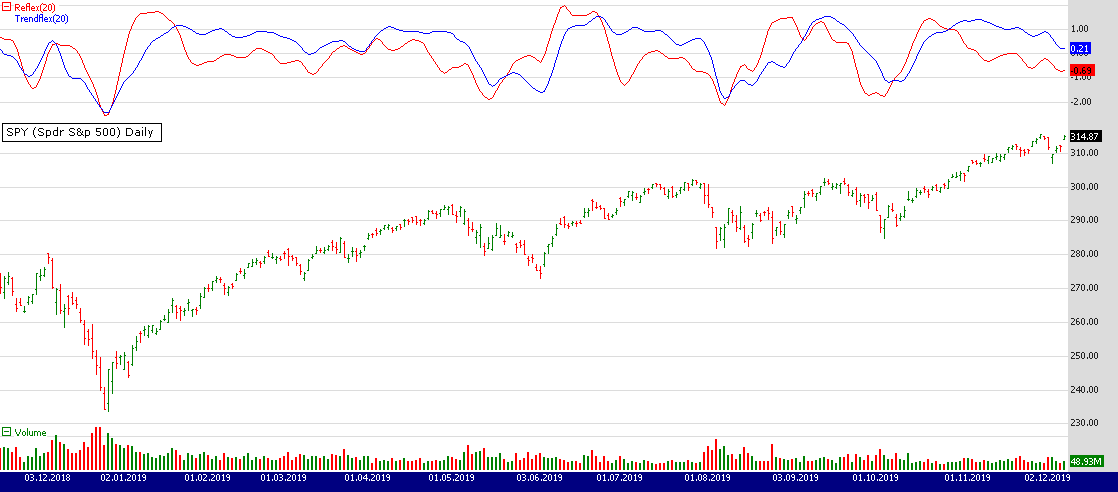FIGURE 3: WEALTH-LAB. The two new indicators, reflex and trendflex, are shown here applied to a daily chart of SPY. (Data provided by Yahoo Finance.)

—Eugene (Gene Geren), Wealth-Lab team
MS123, LLC
www.wealth-lab.com

BACK TO LISTThe reflex indicator and trendflex indicator introduced by John Ehlers in his article in this issue, “Reflex: A New Zero-Lag Indicator,” can be easily implemented in NeuroShell Trader using NeuroShell Trader’s ability to call external dynamic linked libraries. Dynamic linked libraries can be written in C, C++, and Power Basic.

After moving the code given in the article to your preferred compiler and creating a DLL, you can insert the resulting indicator as follows:

1. Select “New Indicator …” from the Insert menu.
2. Choose the External Program & Library Calls category.
3. Select the appropriate External DLL Call indicator.
4. Setup the parameters to match your DLL.
5. Select the finished button.

Users of NeuroShell Trader can go to the Stocks & Commodities section of the NeuroShell Trader free technical support website to download a copy of this or any previous Traders’ Tips.

A sample chart is shown in Figure 4.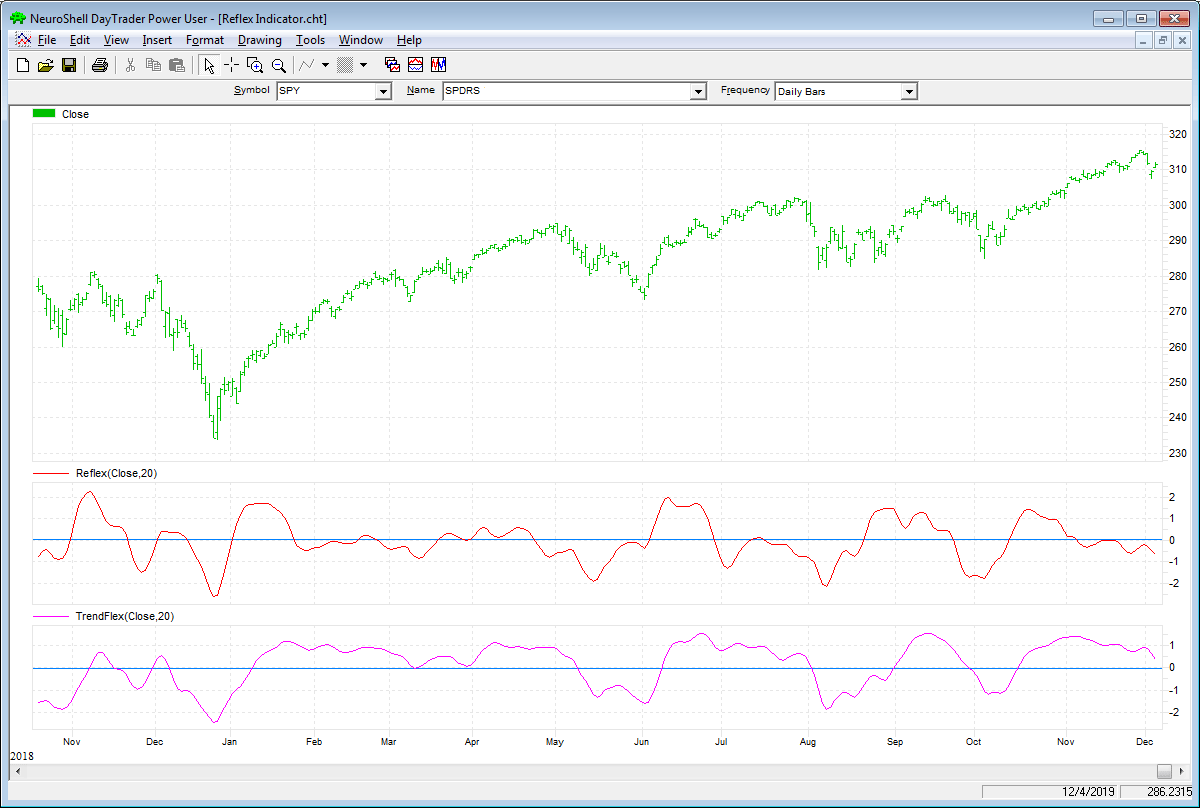FIGURE 4: NEUROSHELL TRADER. This NeuroShell Trader chart shows the reflex and trendflex indicators on a chart of SPY.

—Marge Sherald, Ward Systems Group, Inc.
301 662-7950, sales@wardsystems.com
www.neuroshell.com

BACK TO LIST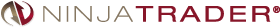The reflex and trendflex indicators discussed in the article in this issue, “Reflex: A New Zero-Lag Indicator” by John Ehlers, are available for download at the following links for NinjaTrader 8 and NinjaTrader 7:

You can review the indicators’ source code in NinjaTrader 8 by selecting the menu New → NinjaScript Editor → Indicators from within the Control Center window and selecting the “reflex” and “trendflex” files. You can review the indicators’ source code in NinjaTrader 7 by selecting the menu Tools → Edit NinjaScript → Strategy from within the Control Center window and selecting the “reflex” and “trendflex” files.

NinjaScript uses compiled DLLs that run native, not interpreted, which provides you with the highest performance possible.

A sample chart implementing the strategy is shown in Figure 5.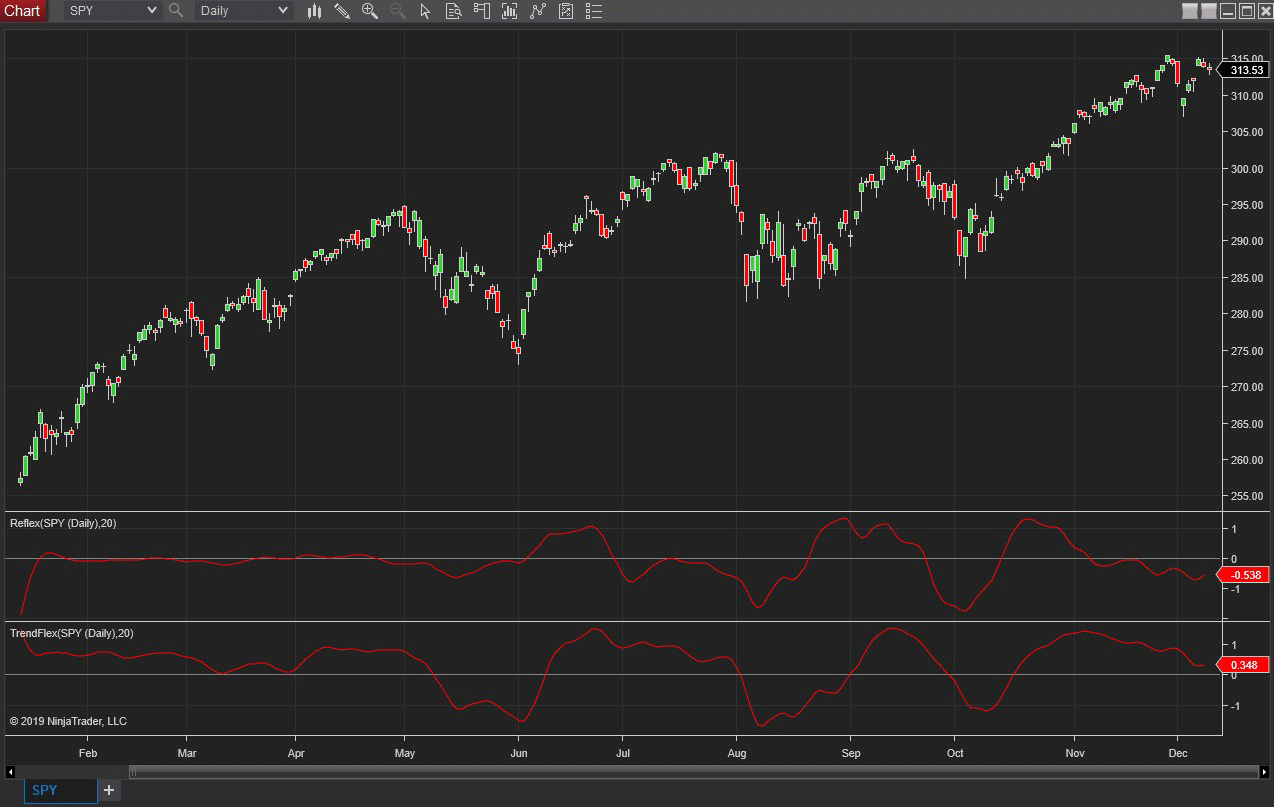FIGURE 5: NINJATRADER. The reflex and trendflex indicators are displayed on a daily SPY chart from January 2019 to December 2019.

—Raymond Deux & Chris Lauber

BACK TO LISTThe importable TradersStudio files based on John Ehlers’ article, “Reflex: A New Zero-Lag Indicator,” can be obtained on request via email to info@TradersEdgeSystems.com.

I coded the indicators described, reflex and trendflex. Figure 6 shows the two indicators with a 20-bar length on a chart of Google during 2011.The code is also shown here:

```'REFLEX: A NEW ZERO-LAG INDICATOR
'Author: John F. Ehlers
'Coded by: Richard Denning 12/15/19

Function EHLERS_REFLEX(Length)
Dim Slope As BarArray
Dim theSum As BarArray
Dim count As BarArray
Dim a1 As BarArray
Dim b1 As BarArray
Dim c1 As BarArray
Dim c2 As BarArray
Dim c3 As BarArray
Dim Filt As BarArray
Dim MS As BarArray
Dim Reflex As BarArray

If BarNumber=FirstBar Then
'Length = 20
Slope = 0
theSum = 0
count = 0
a1 = 0
b1 = 0
c1 = 0
c2 = 0
c3 = 0
Filt = 0
MS = 0
Reflex = 0
End If

a1 = Exp(-1.414*3.14159 / (.5*Length))
b1 = 2*a1*TStation_Cosine(1.414*180 / (.5*Length))
c2 = b1
c3 = -a1*a1
c1 = 1 - c2 - c3
Filt = c1*(Close + Close) / 2 + c2*Filt + c3*Filt
'Length is assumed cycle period
Slope = (Filt[Length] - Filt) / Length
'Sum the differences
theSum = 0
For count = 1 To Length
theSum = theSum + (Filt + count*Slope) - Filt[count]
Next
theSum = theSum / Length
'Normalize in terms of Standard Deviations
MS = .04*theSum*theSum + .96*MS
If MS <> 0 Then
Reflex = theSum / Sqr(MS)
End If
EHLERS_REFLEX = Reflex
End Function
'--------------------------------------------------------------
Function EHLERS_TRENDFLEX(Length)
Dim theSum As BarArray
Dim count As BarArray
Dim a1 As BarArray
Dim b1 As BarArray
Dim c1 As BarArray
Dim c2 As BarArray
Dim c3 As BarArray
Dim Filt As BarArray
Dim MS As BarArray
Dim Trendflex As BarArray

If BarNumber=FirstBar Then
'Length = 20
theSum = 0
count = 0
a1 = 0
b1 = 0
c1 = 0
c2 = 0
c3 = 0
Filt = 0
MS = 0
Trendflex = 0
End If
'Gently smooth the data in a SuperSmoother
a1 = Exp(-1.414*3.14159 / (.5*Length))
b1 = 2*a1*TStation_Cosine(1.414*180 / (.5*Length))
c2 = b1
c3 = -a1*a1
c1 = 1 - c2 - c3
Filt = c1*(Close + Close) / 2 + c2*Filt + c3*Filt
'Sum the differences
theSum = 0
For count = 1 To Length
theSum = theSum + Filt - Filt[count]
Next
theSum = theSum / Length
'Normalize in terms of Standard Deviations
MS = .04*theSum*theSum + .96*MS
If MS <> 0 Then
Trendflex = theSum / Sqr(MS)
End If
EHLERS_TRENDFLEX = Trendflex
End Function
'--------------------------------------------------------------
'Indicator plot for REFLEX:
Sub REFLEX_IND(length)
Dim Reflex As BarArray
Reflex = EHLERS_REFLEX(20)
Plot1(Reflex)
Plot2(0)
End Sub
'---------------------------------------------------------------
'Indicaotr plot for TRENDFLEX:
Sub TRENDFLEX_IND(length)
Dim Trendflex As BarArray
Trendflex = EHLERS_TRENDFLEX(20)
Plot1(Trendflex)
Plot2(0)
End Sub
'--------------------------------------------------------------
Function TSTATION_COSINE(TSdegrees)
End Function
'--------------------------------------------------------------

```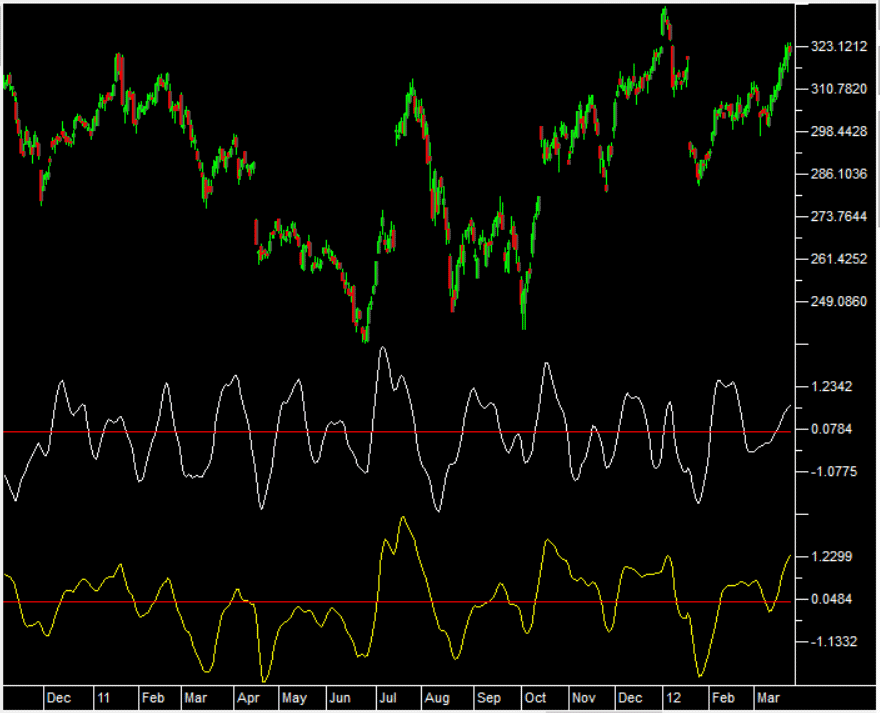FIGURE 6: TRADERSSTUDIO. John Ehlers’ reflex indicator (white) and trendflex indicator (yellow) are shown on a chart of Google during 2011.

—Richard Denning

BACK TO LIST

### MICROSOFT EXCEL: FEBRUARY 2020

In his article in this issue, “Reflex: A New Zero-Lag Indicator,” John Ehlers takes a look at a couple novel indicators with reduced lag.

His reflex indicator is more sensitive to significant reversals, while the trendflex oscillator seems better at recognizing enduring trends. Both seem sensitive to sharper moves relative to recent price behavior.

As a “what if,” I decided to add color bands at the zero crossover points to see if they might be useful as trading signals (see my Figures 8 and 9).

While I did not create a long and short transaction table to verify things, my observations over a few symbols suggest that there might be something to the idea of using the crossovers.

Trendflex is perhaps a bit better for this purpose but would definitely need a few complimentary indicators to confirm entries and exits and to help to reduce whipsaws in the middle of ongoing trends.

It will be well worth your time to look at some other symbols with these two indicators and think about how you might incorporate either or both into your trading toolbox.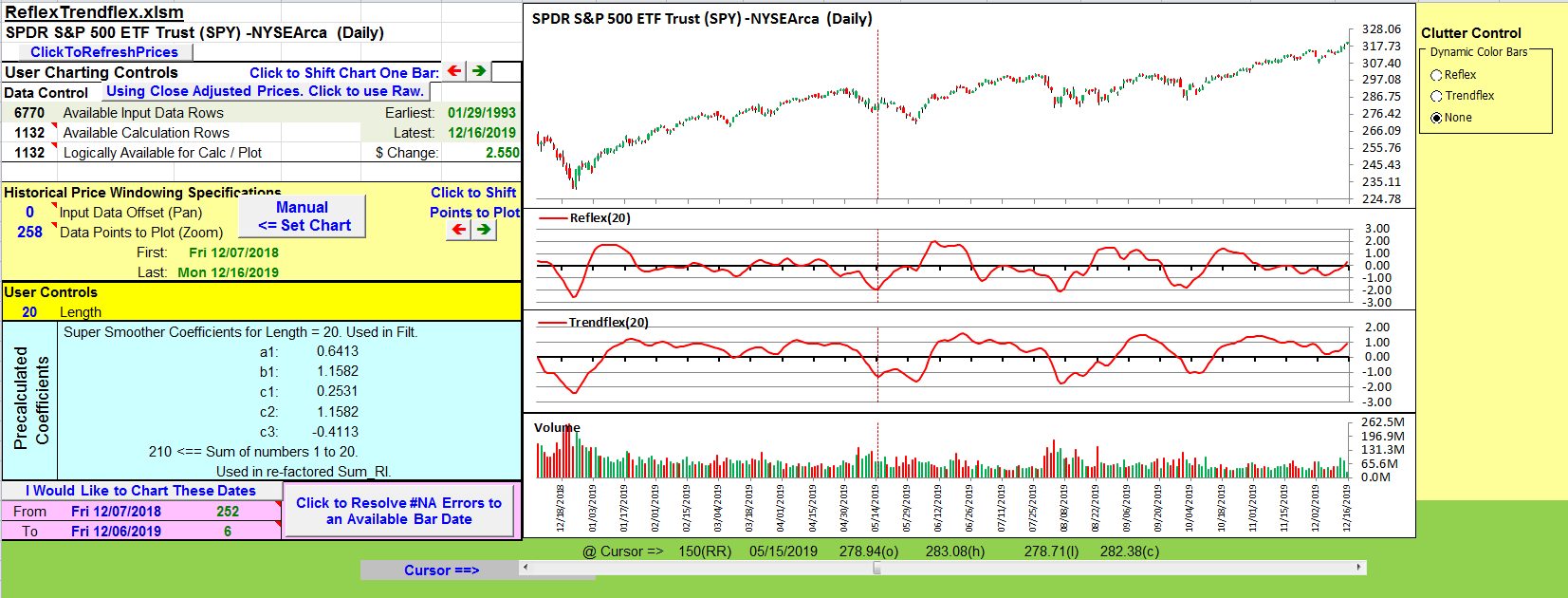FIGURE 7: EXCEL. Reflex and trendflex similar to Figures 3 and 4 from Ehlers’ article.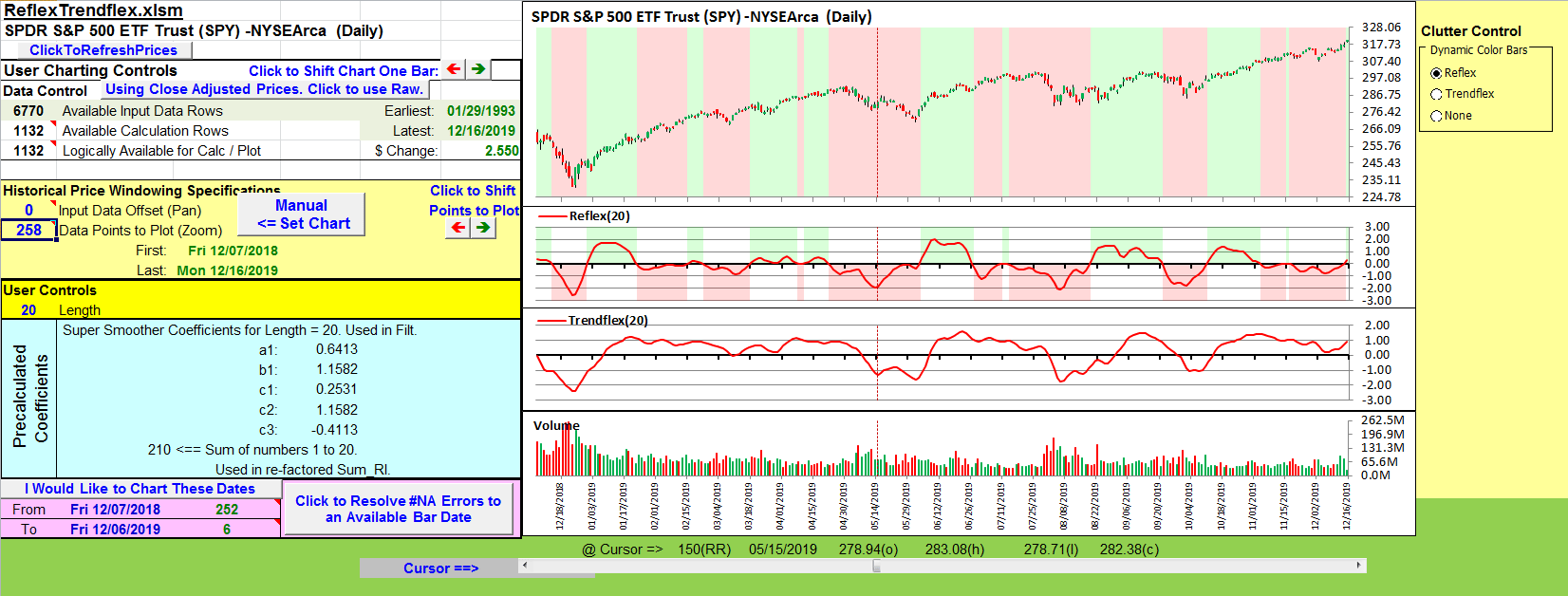FIGURE 8: EXCEL. Color Bands for Reflex Zero Crossovers.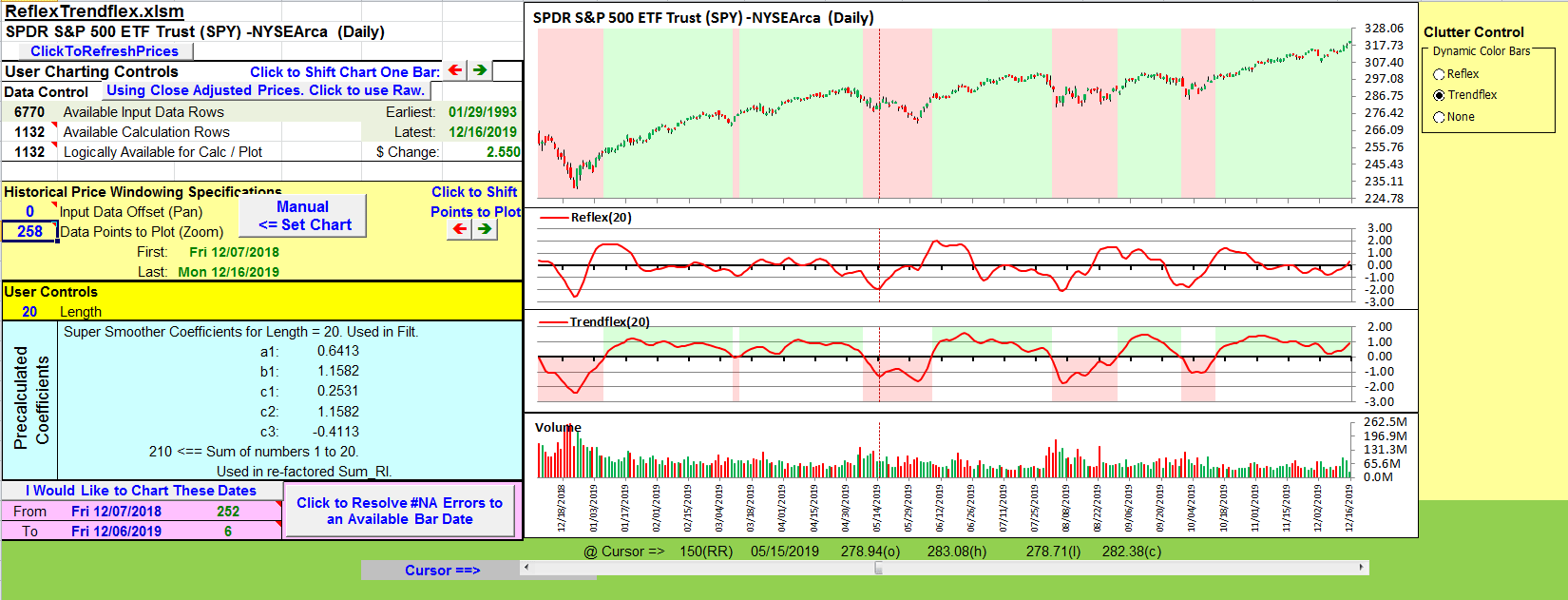FIGURE 9: EXCEL. Color Bands for Trendflex Zero Crossovers.

• Right-click on the Excel file link, then
• Select “save as” (or “save target as”) to place a copy of the spreadsheet file on your hard drive.

—Ron McAllister
Excel and VBA programmer
rpmac_xltt@sprynet.com

BACK TO LIST### THINKORSWIM: FEBRUARY 2020

We have put together a pair of studies based on the article by John Ehlers, “Reflex: A New Zero-Lag Indicator.” We built the studies referenced by using our proprietary scripting language, thinkscript. To ease the loading process, simply click on http://tos.mx/KuKGOLK or enter it into setup—open shared item from within thinkorswim then choose view thinkScript study and name it “reflex.” Do the same with http://tos.mx/aE5oFkr and name it “trendflex.” These can then be added to your chart from the edit study and strategies menu within thinkorswim charts. We should note that we used our ExpAverage() function instead of the simple recursion formula as in the author’s scripts to utilize Prefetch to get better initialization and therefore consistent initial values regardless the chosen time period.

Figure 10 shows these two studies applied to a one-year daily chart of SPY. See John Ehlers’ article for how to interpret these studies.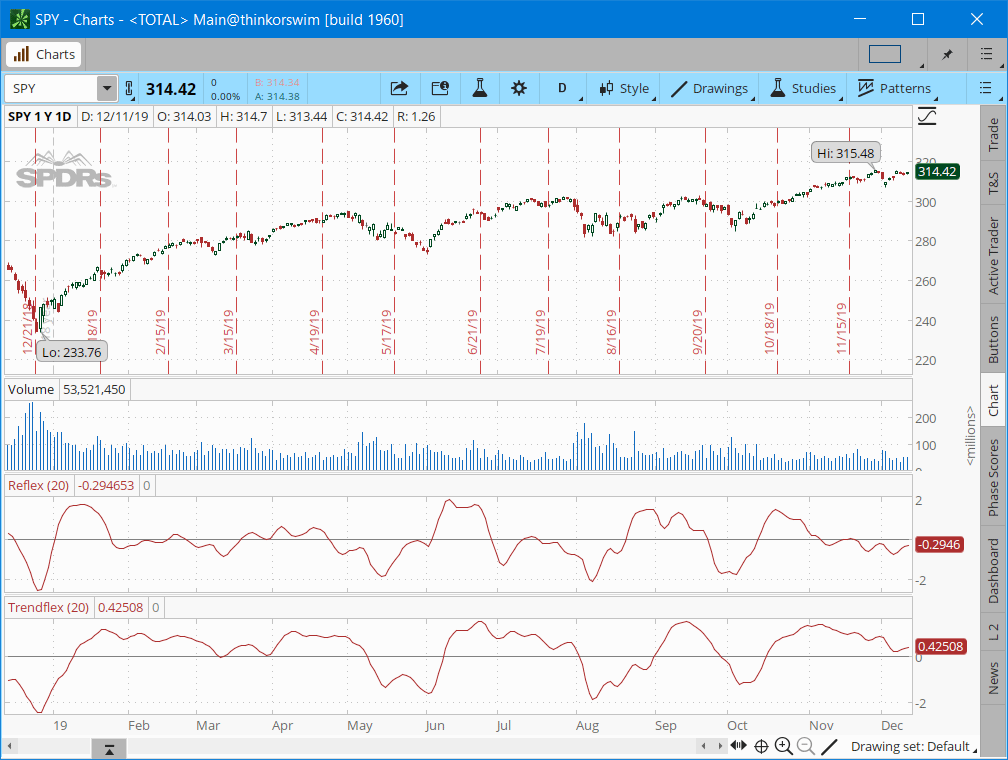FIGURE 10: THINKORSWIM. The two studiesapplied to a one year daily Chart of SPY.

—thinkorswim
A division of TD Ameritrade, Inc.
www.thinkorswim.com

BACK TO LIST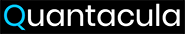### QUANTACULA STUDIO: FEBRUARY 2020

We added the reflex and trendflex indicators to our TASC library for Quantacula, so they are available both on our website and in Quantacula Studio. Here is an example trading strategy written in C# using the reflex indicator. The strategy enters the market when reflex hits a 200-bar low, and exits when it turns down from a 20-bar high. This simple technique is effective at timing entries and exits, as shown in the example chart of VZ in Figure 11.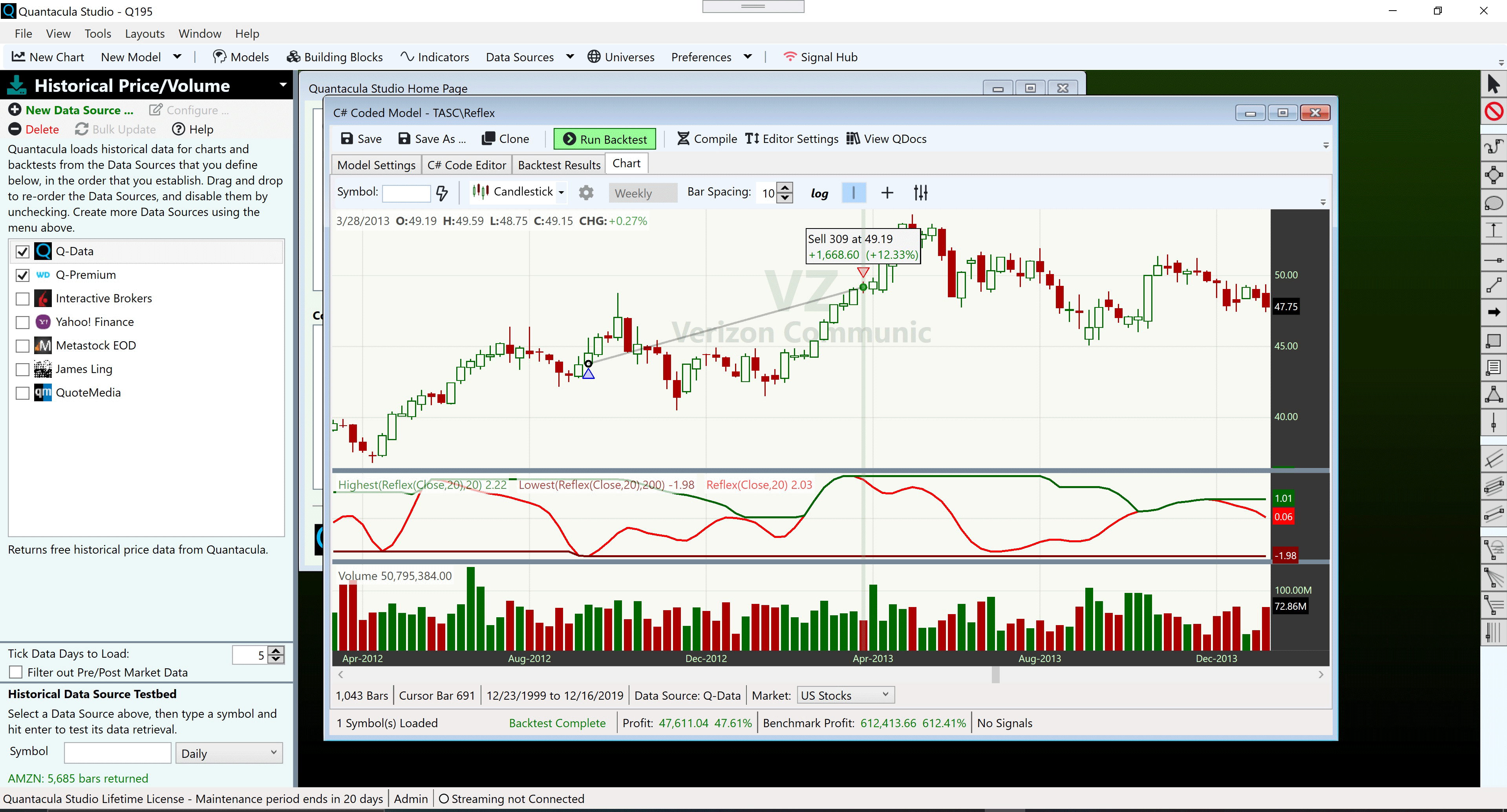FIGURE 11: AN EXAMPLE TRADE IN THE QUANTACULA REFLEX 200/20 STRATEGY

The code is shown here:

```Code:
using QuantaculaBacktest;
using QuantaculaCore;
using QuantaculaIndicators;
using System.Drawing;
using TASCIndicators;

namespace Quantacula
{
public class MyModel1 : UserModelBase
{
//create indicators here
public override void Initialize(BarHistory bars)
{
StartIndex = 200;
reflex = new Reflex(bars.Close, 20);
PlotIndicator(reflex);
reflexLL = new Lowest(reflex, 200);
PlotIndicator(reflexLL, Color.Maroon);
reflexHH = new Highest(reflex, 20);
PlotIndicator(reflexHH, Color.DarkGreen);
}

//execute the strategy rules here
public override void Execute(BarHistory bars, int idx)
{
if (!HasOpenPosition(bars, PositionType.Long))
{
if (reflex[idx] == reflexLL[idx])
{
exitFlag = false;
}
}
else
{
if (exitFlag)
{
if (reflex[idx] < reflexHH[idx])
}
else if (reflex[idx] == reflexHH[idx])
exitFlag = true;
}
}

//declare private variables below
Reflex reflex;
Lowest reflexLL;
Highest reflexHH;
bool exitFlag;
}
}
```

—Dion Kurczek, Quantacula LLC
info@quantacula.com
www.quantacula.com

BACK TO LIST

Originally published in the February 2020 issue of
Technical Analysis of STOCKS & COMMODITIES magazine.